### A.3 Polarization calibration

For polarization observations, the following steps are required. For 21cm or longer wavelength observations, ionospheric Faraday rotation corrections may be needed. See FARAD in the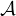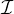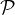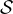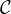ook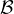ook, but don’t expect much help anymore.

TASAV As added insurance, save your tables again. TGET TASAV; INP; GO.
LISTR Print the parallactic angles of the calibration sources. TGET LISTR; SOUR=’ ’; CALC=’*’; OPTYP=’GAIN’; DPARM=9,0; INP; GO
PCAL Intrinsic antenna polarization calculation. PCAL will be successful only if cal. sources are observed at several parallactic angles. PCAL will modify the AN and SU tables. TASK=’PCAL’; CALS=’cal1’,’cal2’,’ ’; BIF=1; EIF=2; DOCAL=2; REFANT=R; INP; GO
RLDIF Now determine the absolute linear polarization angle. Make a matrix listing of the angle of 3C286. TASK RLDIF’; SOUR=’3C286’,’ ’; DOCAL=2; BIF=1; EIF=0; DOPOL=1;  GAINUSE=2; DOCRT=-1; INP; GO.  The observed angles are different for each frequency and IF . This task returns the average angles for all IFs in CLCORPRM.
CLCOR Now apply the angle corrections to CL table 2. The relative phase of Left and Right circular polarization produces the linear polarization angle and the phase correction is applied to L. The phase difference (twice the angle of linear polarization) for 3C286 is 66o and for 3C138, ϕ = -18o at L band, perhaps -24o at higher frequencies. Change RLDIF’s results to this form with FOR I = 1:20; CLCORP(I)=66-CLCORP(I); END. Then TASK=’CLCOR’; STOKES=’L’; SOUR=’ ’; OPCOD=’POLR’; BIF=1; EIF=2; GAINVER=2; GAINUSE=0; INP; GO.  Run RLDIF again to check the phases.  TGET RLDIF; INP; GO. Note that CLCOR copies the CL table version 2 to 3 while applying the phase correction. If the phases are wrong, delete version 3, return to PCAL and RLDIF and then do another CLCOR.